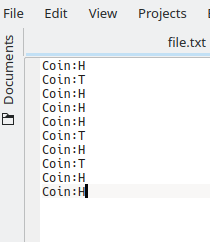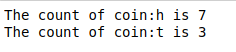# Python program to Count the Number of occurrences of a key-value pair in a text file

• Difficulty Level : Basic
• Last Updated : 29 Dec, 2020

Given a text file of key-value pairs. The task is to count the number of occurrences of the key-value pairs in the file.

Examples:

```Input File:
Coin:H
Coin:T
Coin:H
Coin:H
Coin:H
Coin:T
Coin:H
Coin:T
Coin:H
Coin:H

Output:
The count of coin:h is 7
The count of coin:t is 3

Input File:
geeks:G
for:F
geeks:G
geeks:G
geek:H
for:F
geek:H

Output:
The count of geeks:g is 3
The count of for:f is 2
The count of geek:h is 2
```

The approach is very simple. Maintain another dictionary (say d) that will store the count of occurrence of each key-value pair of the file. Store the key-value pair of the text file as a key in the dictionary. Now iterate through the key-value pairs of the file. If the pair is present in the dictionary then increment the value of that pair by one otherwise insert the pair and set its value to one.

Below is the implementation.

Text file:`# Python program to count the ` `# occurrences of key-value pair ` `# in the text file ` ` `  ` `  `# opening text file ` `f ``=` `open``(``"file.txt"``, ``"r"``) ` `d ``=` `dict``() ` ` `  `for` `res ``in` `f: ` `    ``# removing new line and extra ` `    ``# space characters ` `    ``res ``=` `res.strip() ` ` `  `    ``# changing ase to prevent matching ` `    ``# errors ` `    ``res ``=` `res.lower() ` ` `  `    ``# separating key-value pairs ` `    ``lines ``=` `res.split() ` ` `  `    ``for` `line ``in` `lines: ` ` `  `        ``if` `line ``in` `d: ` ` `  `            ``# If the key-value pair ` `            ``# is present in d then  ` `            ``# increment its value by one ` `            ``d[line] ``=` `d[line]``+``1` `        ``else``: ` ` `  `            ``# Insert the key-value pair ` `            ``# in the dictionary and sets ` `            ``# its value to one ` `            ``d[line] ``=` `1` ` `  `f.close() ` ` `  `# Printing Result ` `for` `key ``in` `list``(d.keys()): ` `    ``print``(``"The count of {} is {}"``.``format``(key,d[key])) `

Output :My Personal Notes arrow_drop_up
Recommended Articles
Page :